## Classification of Pulse Signals:

The Classification of Pulse Signals are divided into four categories, namely

1. Periodic signals.
2. Aperiodic signals.
3. Random signals.
4. Continuous and Discrete Signals

### 1. Periodic Signals:

Periodic signals are those which repeat themselves at regular intervals of time say ‘T’. This time ‘T’ is referred to as the time period. Sinusoidal signals, time-base signals and rectified waveforms (i.e., the output of the rectifier circuits) etc. fall under this category of pulse signals.

A periodic signal may be expressed as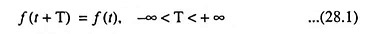where T is the time period.

Repetition frequency fR of a periodic signal is the reciprocal of the time period T. Thus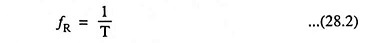By iteration of Eq. (28.1) we have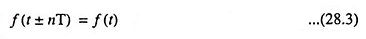where n is any integer i.e., n = 1, 2, 3 ….

The average value or mean value of a repetitive signal of periodic time T is given by the following expression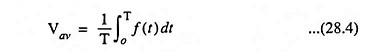The  rms value of a periodic or repetitive signal is given asThe instantaneous power, p is defined as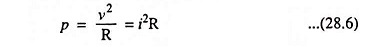where v is the instantaneous voltage, i is the instantaneous current and R is the circuit resistance.

Thus average power of a voltage pulse is given as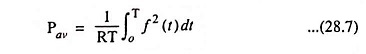Combining Eqs. (28.5) and (28.7) we have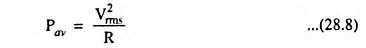### 2. Aperiodic Signals:

Aperiodic signals are those which occur only once in the entire time period (i.e., in the time interval from -∞ to +∞). Thus an aperiodic signal may be considered to be a periodic signal of infinite time period. Transients of different form constitute aperiodic signals. Since the aperiodic signals last for a short duration of time, our interest lies in storing it for analysis purpose. This may he accomplished by digital techniques i.e., amplitude and time quantization and digital storage.

### 3. Random Signals:

Random signals occur without any well defined mathematical description. At most such signals can be described by probabilistic models. Noise is one such random signal. A class of random signals which find applications in digital electronics is binary random signals. It is a two-valued random signal with random or unpredictable zero crossings. An example is a telegraph signal.

### 4. Continuous and Discrete Signals:

Pulse signals may be of two types, viz.; (i) continuous signal and (ii) discrete in time.

A continuous signal is one which is continuous function of independent variable “t” such as a triangular waveform or a staircase waveform, as depicted in Figs. 28.1 (a) and 28.1 (b) respectively. The signal must uniquely be defined at all values of time “t” within a given time range.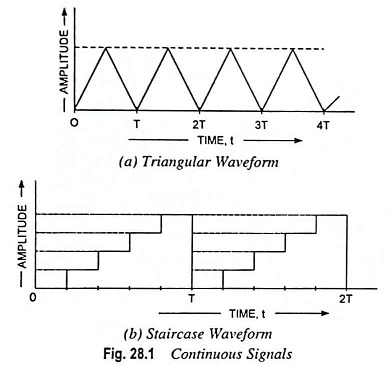Discrete signal is defined only at a sequence of discrete val­ues of independent variable “t”. In many practical situations the instants at which signals are defined are equally spaced and may be defined by t = t± nτ where n takes only integral values (i.e., n = 1, 2, 3, …) and τ is the time duration between instants.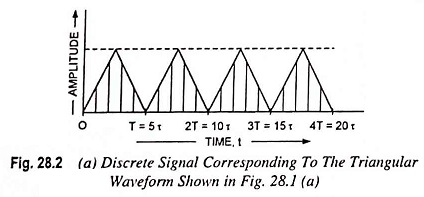It is also possible to recover the original continuous signal from dis­crete signal. Discrete signals find wide applications in digital in­strumentation, digital control systems and communications.

Scroll to Top